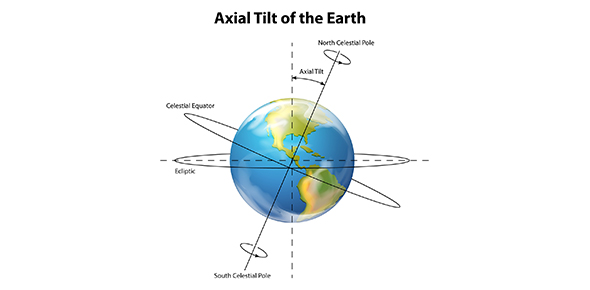# Quiz: Earth Science Multiple Choice Test

20 Questions | Total Attempts: 1175SettingsEarth science gives us a new way to learn more about the universe we exist in. Having gathered a lot of knowledge in your past classes, you are seen as capable of answering the quiz below. Give it a try and polish up the ones you do not get right.

• 1.
32,000 is written in scientific notation as:
• A.

A. 3.2x10^4

• B.

B. 32x10^4

• C.

C. 32x10^3

• D.

D. 3.2x10^3

• 2.
What type of star system has no defined shape?
• A.

A. Irregular Galaxy

• B.

B. Milky Way

• C.

C. Spiral Galaxy

• D.

D. Binary Star System

• 3.
A binary star system has more than three stars.
• A.

True

• B.

False

• 4.
What is an eclipsing binary?
• A.

A. Another name for a binary star system

• B.

B. A binary star system in which one star periodically blocks the other's light

• C.

C. When the moon eclipses the sun

• D.

D. When the sun eclipses the moon

• 5.
What is inertia?
• A.

A. The tendency of an object to resist change in motion

• B.

B. How fast an object is going divided by the distance

• C.

C. Mass of an object divided by its volume

• D.

D. A type of gas for which the inert gases were named

• 6.
What is a neap tide?
• A.

A. A tide that occurs when the difference between highest and lowest tides is least

• B.

B. A tide that occurs when the difference between highest and lowest tides is the most

• C.

C. A tide that occurs every 100 years

• D.

D. A tide that occurs whenever there is a full moon two months in a row.

• 7.
What is a spring tide?
• A.

A. A tide that occurs when the difference between highest and lowest tides is the least

• B.

B. A tide that occurs when the difference between highest and lowest tides is the most

• C.

C. A tide that occurs every 100 years

• D.

D. A tide that occurs whenever there is a full moon three months in a row

• 8.
How are tides created?
• A.

A. Based on the moon's position

• B.

B. Based on the Earth's axis

• C.

C. Based on the Earth's tilt

• D.

D. Based on the height of the usn in the sky

• 9.
What does AU stand for in Spacial Sciences?
• A.

A. Gold

• B.

B. Astronomical Unit

• C.

C. Auhsfart

• D.

D. Atoms United

• 10.
What is a light year?
• A.

A. How many earth years it takes light to reach the sun.

• B.

B. The distance that light travels in one year

• C.

C. A small part of a year

• D.

D. The distance that light travels while on its journey to reach the moon

• 11.
The four gas giants are: Mercury, Venus, Earth, and Mars.
• A.

True

• B.

False

• 12.
The four terrestrial planets are: Mercury, Venus, Earth, and Mars.
• A.

True

• B.

False

• 13.
There are 9 planets.
• A.

True

• B.

False

• 14.
A space spinoff is
• A.

A. When 2 spaceships spin in space

• B.

B. Something developed for use in space that has uses on Earth

• C.

C. Something developed for use on Earth that has uses in space

• D.

D. When a spacecraft crashes before entering orbit

• 15.
A space probe can hold a manned space crew.
• A.

True

• B.

False

• 16.
A space ship can hold a manned crew.
• A.

True

• B.

False

• 17.
What is a rocket?
• A.

A. A missile

• B.

B. A toy

• C.

C. Something that exerts gas in one direction to move the other direction

• D.

D. Something that exerts gas in the direction that it is trying to move in

• 18.
The moon is Earth's natural satellite.
• A.

True

• B.

False

• 19.
A planet can have more than one moon.
• A.

True

• B.

False

• 20.
What is a satellite?
• A.

A. Something that brings us cable

• B.

B. A device put in space to orbit another object

• C.

C. Something that can only be controlled by someone in space

• D.

D. A device that only works on Saturdays

Related TopicsBack to top